#2018 iT 邦幫忙鐵人賽DAY 12
3
AI & Machine Learning

## Day 12：物體偵測(Object Detection) + 影像標題(Image Captioning)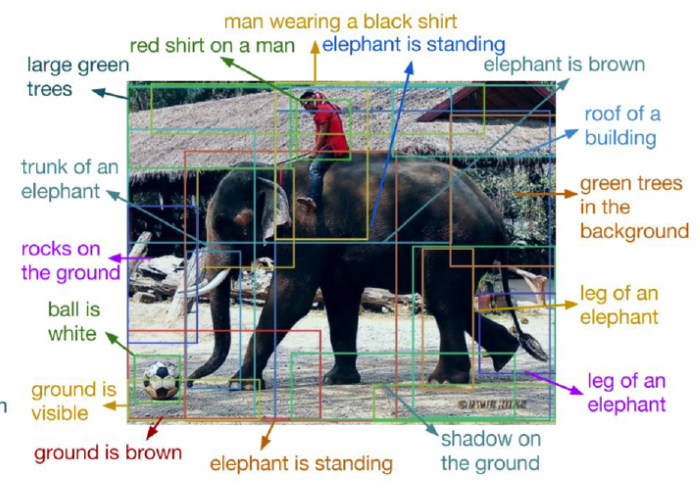## 前言

1. 分類(Classification)
2. 按語意切割(Semantic Segmentation)：按事物類別區分像素分塊，不區分『實例』(Instance)。
3. 定位(Classification + Localization)：標註單一物體(Single Object)所在的位置及大小。
4. 物體偵測(Object Detection)：標註多個物體(Multiple Object)所在的位置及大小。
5. 實體切割(Instance Segmentation)：標註『實例』(Instance)，同一類的物體可以區分各別的位置及大小，尤其物體之間有重疊。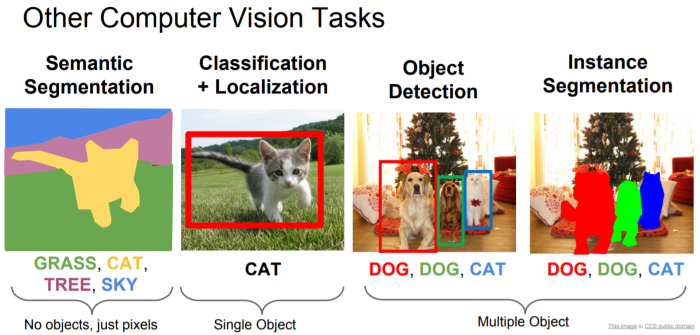圖. 除了分類(Classification)，其他電腦視覺的應用，圖片來源：cs231n_2017_lecture11 Detection and Segmentation

## 相關技術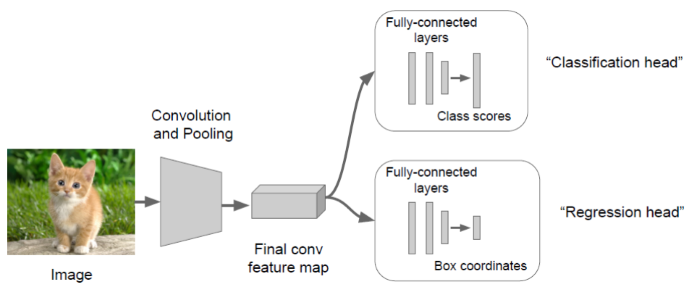1. 滑動視窗(Sliding Window)：這是一種窮舉法，設定各種尺寸的區域，從左上角開始滑動，找出所有區域，然後，看哪一個區域符合的機率最高。這種方法最簡單，但是也相對耗時。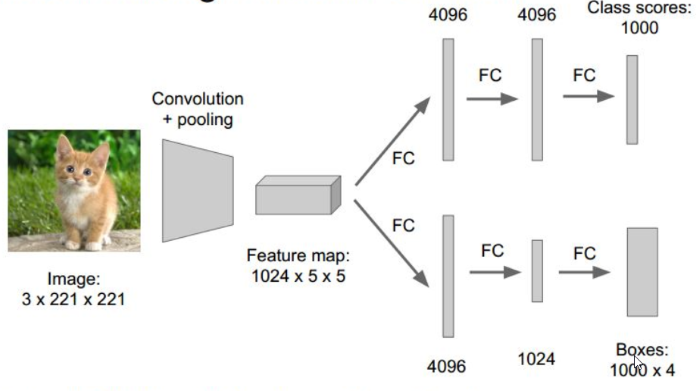圖. 滑動視窗(Sliding Window)模型，圖片來源：CS231n第八課：目標檢測定位學習記錄 - IT閱讀

2. Region Proposals：利用了圖像中的紋理、邊緣、顏色等信息，人為定義可能含有目標的RoI(Regions of Interest)區域，只針對這些區域比較符合的機率，每秒可過濾上千個區域，相關演算法有 R-CNN、Fast R-CNN、Faster R-CNN...等。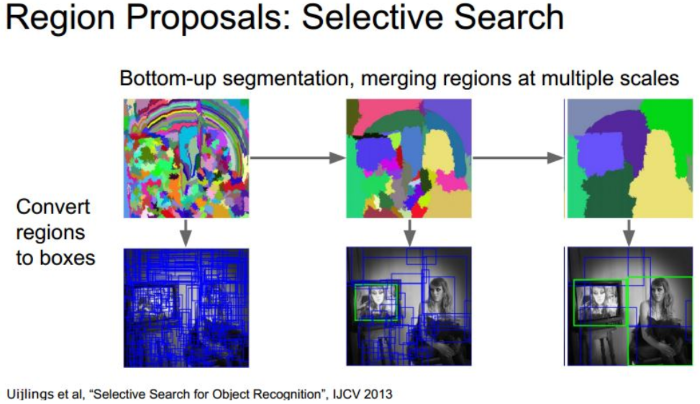圖. Region Proposals 示意圖，圖片來源：CS231n第八課：目標檢測定位學習記錄 - IT閱讀

3. 迴歸方法：將圖片切成小方塊(Grid)，再以小方塊中心點選擇幾種尺寸的區域，利用迴歸方法計算每個區域含有目標的機率。這種方法較 Region Proposals 慢，但適合即時(Real Time)偵測，目前相關演算法有有 YOLO(You Only Look Once)、SSD(Single Shot MultiBox Detector)...等。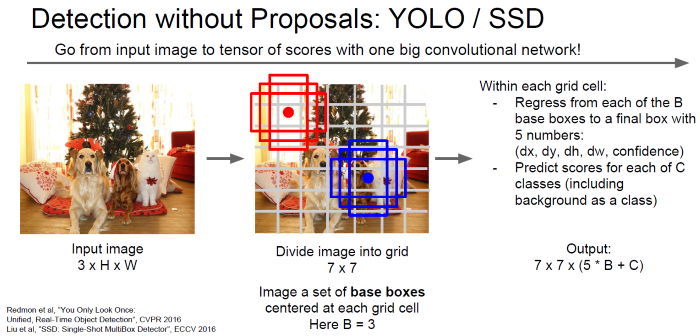圖. 迴歸方法說明，圖片來源：cs231n_2017_lecture11 Detection and Segmentation

## 實作

python ssd_test.py

``````"""
Run object detection with VOC classes.

This is just a minor modification of code from
https://github.com/rykov8/ssd_keras
"""

from keras.applications.imagenet_utils import preprocess_input
from keras.preprocessing import image
import matplotlib.pyplot as plt
import numpy as np
from scipy.misc import imread
import sys

from ssd import SSD300
from ssd_utils import BBoxUtility
import os
from os.path import basename

def create_overlay(img, results, , plt_fname):
plt.clf()
# Parse the outputs.
det_label = results[:, 0]
det_conf = results[:, 1]
det_xmin = results[:, 2]
det_ymin = results[:, 3]
det_xmax = results[:, 4]
det_ymax = results[:, 5]

# Get detections with confidence higher than 0.6.
top_indices = [i for i, conf in enumerate(det_conf) if conf >= 0.6]

top_conf = det_conf[top_indices]
top_label_indices = det_label[top_indices].tolist()
top_xmin = det_xmin[top_indices]
top_ymin = det_ymin[top_indices]
top_xmax = det_xmax[top_indices]
top_ymax = det_ymax[top_indices]
colors = plt.cm.hsv(np.linspace(0, 1, 21)).tolist()

plt.imshow(img / 255.)
currentAxis = plt.gca()
currentAxis.axis('off')

for i in range(top_conf.shape):
xmin = int(round(top_xmin[i] * img.shape))
ymin = int(round(top_ymin[i] * img.shape))
xmax = int(round(top_xmax[i] * img.shape))
ymax = int(round(top_ymax[i] * img.shape))
score = top_conf[i]
label = int(top_label_indices[i])
label_name = voc_classes[label - 1]
display_txt = '{:0.2f}, {}'.format(score, label_name)
coords = (xmin, ymin), xmax - xmin + 1, ymax - ymin + 1
color = colors[label]
currentAxis.add_patch(plt.Rectangle(*coords,
fill=False,
edgecolor=color,
linewidth=2))
currentAxis.text(xmin, ymin, display_txt,
bbox={'facecolor': color, 'alpha': 0.5})
plt.savefig(plt_fname)
print("save "+plt_fname)

if __name__ == "__main__":
import glob
imagesList = glob.glob("images/*.jpg")

# Load the model
voc_classes = ['Aeroplane', 'Bicycle', 'Bird', 'Boat', 'Bottle',
'Bus', 'Car', 'Cat', 'Chair', 'Cow', 'Diningtable',
'Dog', 'Horse', 'Motorbike', 'Person', 'Pottedplant',
'Sheep', 'Sofa', 'Train', 'Tvmonitor']
NUM_CLASSES = len(voc_classes) + 1
input_shape = (300, 300, 3)
model = SSD300(input_shape, num_classes=NUM_CLASSES)
model.load_weights('weights_SSD300.hdf5', by_name=True)
bbox_util = BBoxUtility(NUM_CLASSES)

# Load the inputs
inputs = []
images = []
for img_path in imagesList:
print("process " + img_path)
img = image.load_img(img_path, target_size=(300, 300))
img = image.img_to_array(img)
images.append(imread(img_path))
inputs.append(img.copy())
# 前置處理
print("前置處理...")
inputs = preprocess_input(np.array(inputs))

# 預測
print("預測...")
preds = model.predict(inputs, batch_size=1, verbose=1)
# 取得預測結果
results = bbox_util.detection_out(preds)
print("results=")
print(results)

# create folder if not exist
output_directory="results"
if not os.path.exists(output_directory):
os.makedirs(output_directory)
# proces images
for i, img in enumerate(images):
# 產生有框的 output files
create_overlay(img, results[i], voc_classes,
output_directory+"/{}.png".format(basename(os.path.splitext(imagesList[i]))))
# Garbage collection, to prevent from TensorFlow error
import gc
gc.collect()
``````

## 程式說明

1. 首先會搜尋images資料夾下的jpg檔案。
2. 呼叫 SSD300 函數，載入模型及訓練好的參數檔。
3. 呼叫 detection_out 函數，取得預測結果，放在 results 變數，內容包括的類別序號及對應的機率，還有標註方框的位置及大小。
4. 呼叫 create_overlay 函數，將標註方框及類別名稱畫在原圖上，另存新檔，放在 results 資料夾，附檔名為 png。
5. SSD 模型定義在 ssd.py 中，該模型結構非常複雜，可以在呼叫 SSD300 函數後，加下列指令將結構圖存檔：
``````from keras.utils.vis_utils import plot_model
plot_model(model, to_file='model_plot.png', show_shapes=True, show_layer_names=True)
``````

1. 影像標題(Image Captioning)：結果不只會有方框，也會標註類別名稱，如果不在 20 類的物體會顯示錯誤的類別名稱，如大象及熊讚。
2. 只限20類是因為我們直接載入訓練好的模型(Pre-trained Model) -- weights_SSD300.hdf5，它的訓練資料只含20類物體，實際應用時，可以針對目標，放入更適合的類別資料，重新訓練，就可以得到我們想要的結果了。
3. 訓練資料來源為PASCAL Visual Object Classes
4. 理論基礎請參考『SSD: Single Shot MultiBox Detector』。
5. 本例是將結果存檔，如果要將結果直接顯示出來，可搭配使用 OpenCV 工具箱。

## 結語

### 4 則留言

1

model.load_weights('weights_SSD300.hdf5', by_name=True)

"再將訓練資料集放入模型中，重新訓練即可。"

model.load_weights('weights_SSD300.hdf5', by_name=True)

train_history = model.fit(x=x_Train_norm, y=y_TrainOneHot, validation_split=0.2, epochs=10, batch_size=800, verbose=2)

0

Keras 的程序是固定的

1. 建立模型：本例如下，SSD300 定義在 ssd.py
model = SSD300(input_shape, num_classes=NUM_CLASSES)

2. 訓練模型：model.fit，本例直接載入事先訓練的參數(model.load_weights)，未進行訓練，故要自行訓練，則需改為fit

3. 評估模型：model.evaluate

4. 預測：model.predict

day2 展示自行訓練的完整程序，所以，本例要自行訓練，可參考day2。

from keras.models import load_model
model.save('model.h5') # creates a HDF5 file 'model.h5'

peterhsu iT邦新手 5 級 ‧ 2018-01-23 19:15:52 檢舉

0

0

ssd_test.py 第90行
img = image.load_img(img_path, target_size=(300, 300))